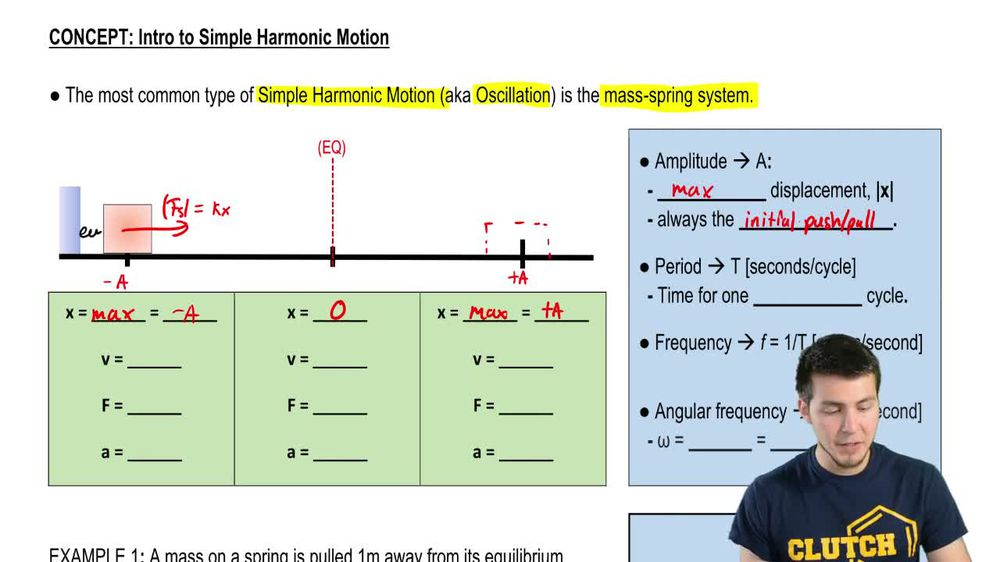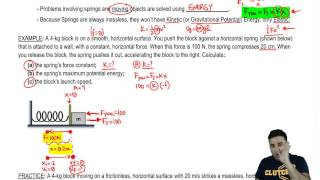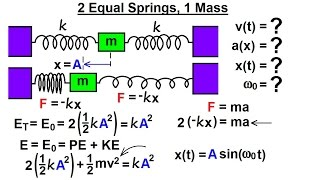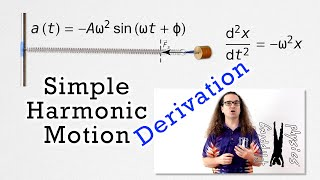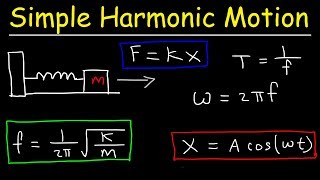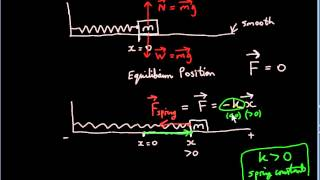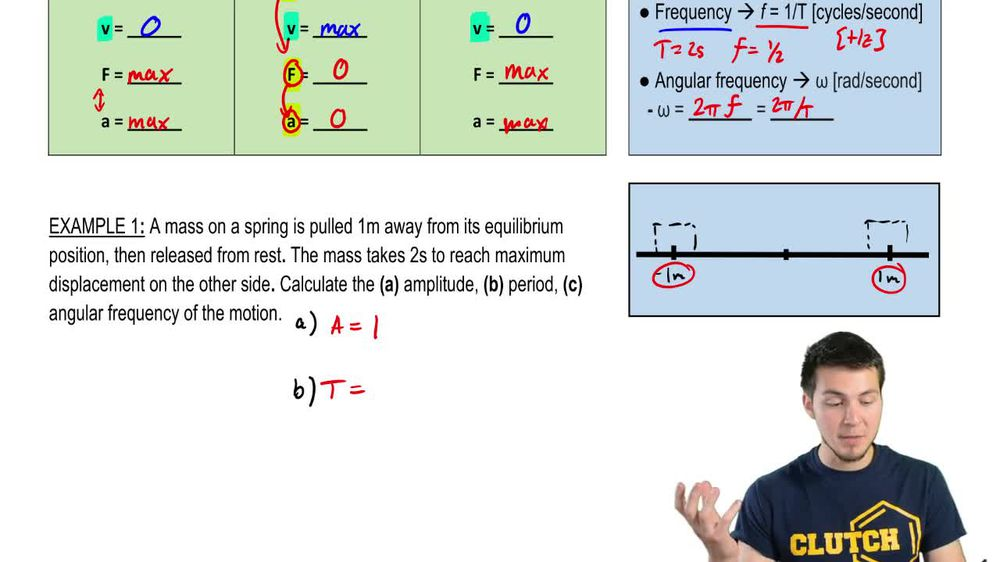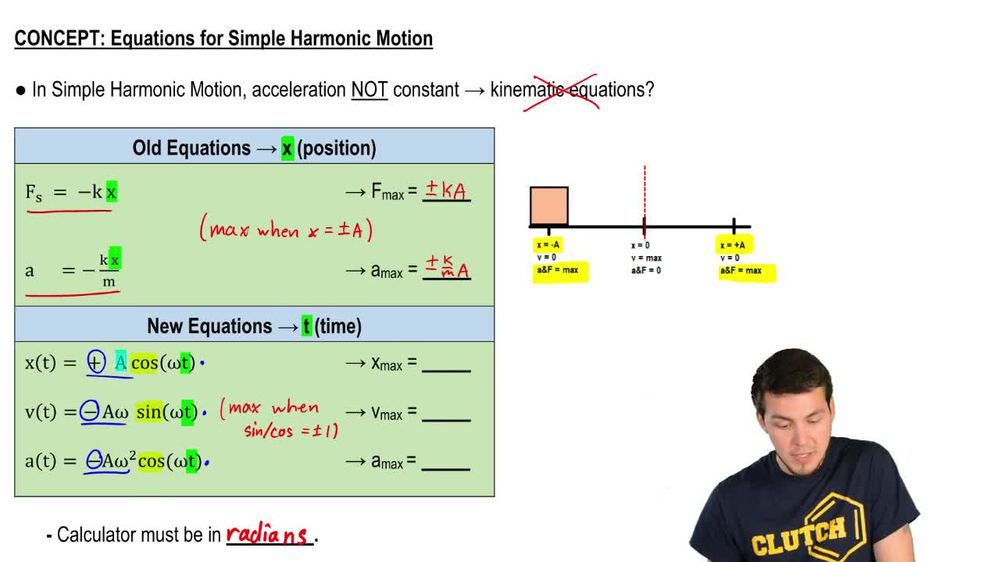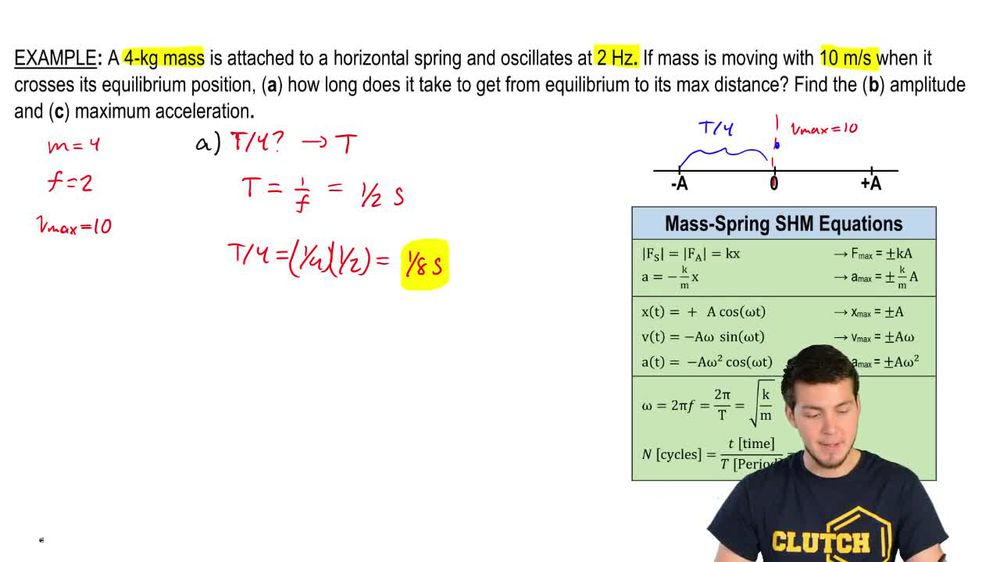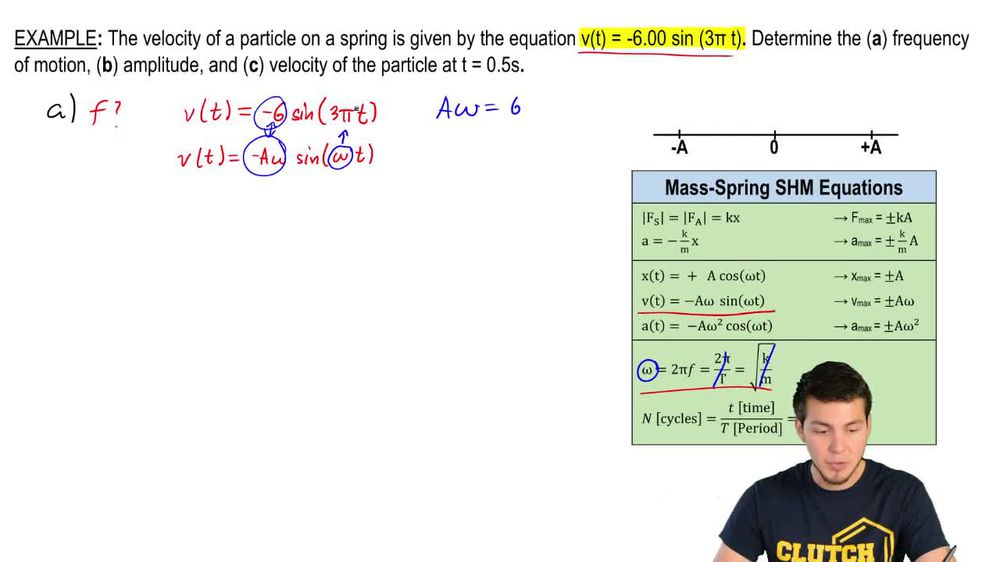Start typing, then use the up and down arrows to select an option from the list.
1. 17. Periodic Motion2. Intro to Simple Harmonic Motion (Horizontal Springs)# Example

by Patrick Ford
162 views
3
3
Hey, guys, let's take a look at this. This example here. We've got a mass on a spring and it's pulled 1 m away from its equilibrium position. So we've got 1 m and we're just gonna draw a little box here, and then you release it from rest. But this is a mass spring system, right? So it's just gonna go and reach the other side where now the distance here, the displacement is gonna be negative 1 m, and that's just gonna go back and forth forever. So here in this first part were asked to calculate the amplitude. But we know that the amplitude is just the maximum displacement on either side. So that means that the amplitude of this is just 1 m. So the second question now were asked to find the period. So that is going to be that letter t. So what is the period look like? What we're told that the mass takes two seconds to reach the maximum displacement on the other side. So if you release it over here, then this time that it takes for you to go all the way over to the other side was equal to two seconds. Now the question is is that the whole period? No, it's not because we said that the period is equal to the time that it takes you to complete one whole entire cycle here. So this two seconds really only represents Ah, half period. This thing has to go all the way back to the other side for another two seconds. And that's gonna be another half period. Which means that these points in between here are actually quarter periods. Right, So this is a quarter, This is a quarter. These are all quarter periods, and this is the smallest sort of division that you could make. So you've got half periods and quarter periods, so we're told that it's two seconds to get to the other side, which means that the full period of this motion is going to be four seconds. Now we've got in this third part. Here is the angular frequency off the motion. So how do we relate angular frequency to the period? Well, we've got an equation appeared that could do that. So if we're looking for the angular frequency Omega we can use is either two pi times the frequency or we can use to pie divided by the period which we actually know. So I'm just gonna go ahead and use that I've got omega equals two pi divided by the full period of cycle, which is for and what you should get is 1 57. And that's gonna be radiance per second. So that's it for this one. Let's keep going with some more examples.